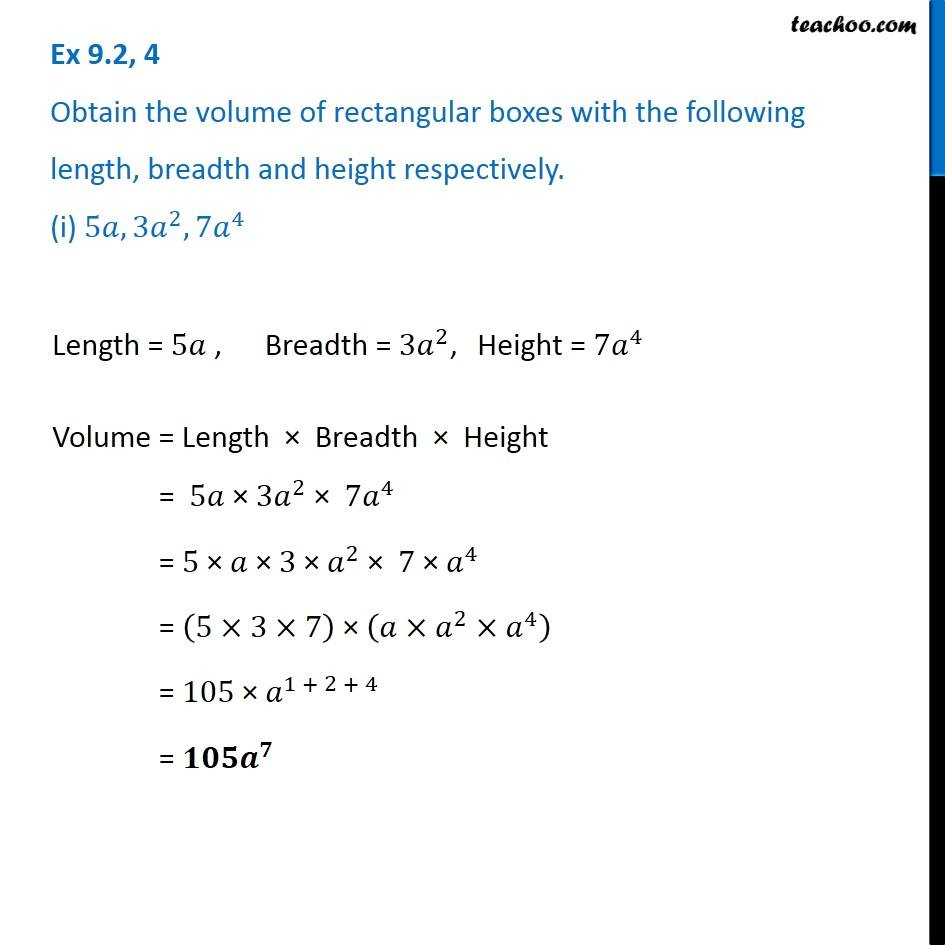Multiplication of Monomials

Chapter 8 Class 8 Algebraic Expressions and Identities
Concept wiseLearn in your speed, with individual attention - Teachoo Maths 1-on-1 Class

### Transcript

Ex 8.2, 4 Obtain the volume of rectangular boxes with the following length, breadth and height respectively. (i) 5𝑎, 3𝑎^2, 7𝑎^4 Length = 5𝑎 , Breadth = 〖3𝑎〗^2, Height = 〖7𝑎〗^4 Volume = Length × Breadth × Height = 5𝑎 × 〖3𝑎〗^2 × 〖7𝑎〗^4 = 5 × 𝑎 × 3 × 𝑎^2 × 7 × 𝑎^4 = (5×3×7) × (𝑎×𝑎^2×𝑎^4 ) = 105 × 𝑎^(1 + 2 + 4) = 𝟏𝟎𝟓𝒂^𝟕